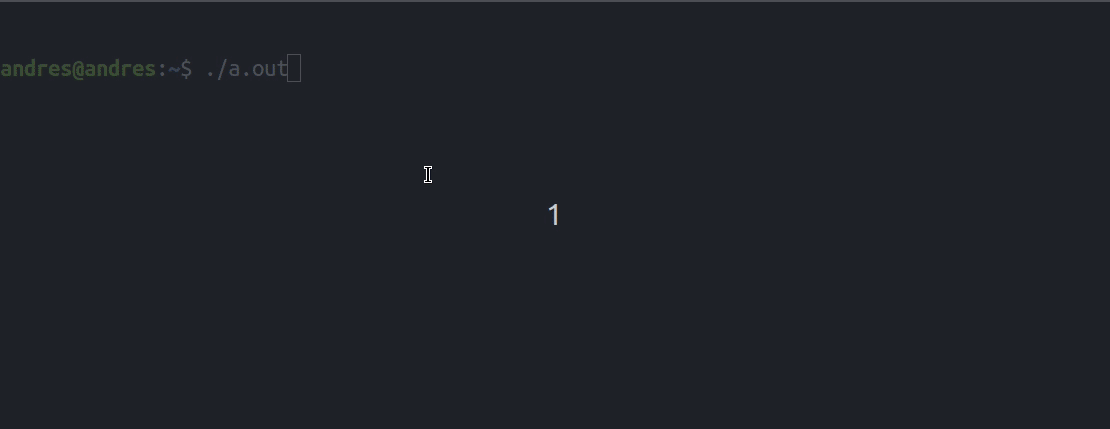# Timer in C++ using system calls

The task is to create timer without using any graphics or animation. The timer will be made using system calls whereever necessary. Timer in this context means a stopwatch with up-counting of time.

The timer is created in Linux. Following system calls of Linux are used:
sleep() : It will make the program sleep for number of seconds provided as arguments to the function.
system() : It is used to execute a system command by passing the command as argument to this function.

## Recommended: Please try your approach on {IDE} first, before moving on to the solution.

Below is the implementation for creating timer using System Calls:

 `// CPP program to create a timer ` `#include ` `#include ` `#include ` `#include ` `using` `namespace` `std; ` ` `  `// hours, minutes, seconds of timer ` `int` `hours = 0; ` `int` `minutes = 0; ` `int` `seconds = 0; ` ` `  `// function to display the timer ` `void` `displayClock() ` `{ ` `    ``// system call to clear the screen ` `    ``system``(``"clear"``); ` ` `  `    ``cout << setfill(``' '``) << setw(55) << ``"         TIMER         \n"``; ` `    ``cout << setfill(``' '``) << setw(55) << ``" --------------------------\n"``; ` `    ``cout << setfill(``' '``) << setw(29); ` `    ``cout << ``"| "` `<< setfill(``'0'``) << setw(2) << hours << ``" hrs | "``; ` `    ``cout << setfill(``'0'``) << setw(2) << minutes << ``" min | "``; ` `    ``cout << setfill(``'0'``) << setw(2) << seconds << ``" sec |"` `<< endl; ` `    ``cout << setfill(``' '``) << setw(55) << ``" --------------------------\n"``; ` `} ` ` `  `void` `timer() ` `{ ` `    ``// infinte loop because timer will keep  ` `    ``// counting. To kill the process press ` `    ``// Ctrl+D. If it does not work ask ` `    ``// ubuntu for other ways. ` `    ``while` `(``true``) { ` `         `  `        ``// display the timer ` `        ``displayClock(); ` ` `  `        ``// sleep system call to sleep  ` `        ``// for 1 second ` `        ``sleep(1); ` ` `  `        ``// increment seconds ` `        ``seconds++; ` ` `  `        ``// if seconds reaches 60 ` `        ``if` `(seconds == 60) { ` `         `  `            ``// increment minutes ` `            ``minutes++; ` ` `  `            ``// if minutes reaches 60 ` `            ``if` `(minutes == 60) { ` `         `  `                ``// increment hours ` `                ``hours++; ` `                ``minutes = 0; ` `            ``} ` `            ``seconds = 0; ` `        ``} ` `    ``} ` `} ` ` `  `// Driver Code ` `int` `main() ` `{ ` `    ``// start timer from 00:00:00 ` `    ``timer(); ` `    ``return` `0; ` `} `

Output:Note: This can be made to run on Windows with a bit of modifications.
Modifications required:
1. Use “cls” in place of “clear” in system() call.
2. Use ‘S’ in sleep() function in place of lower case ‘s’ in sleep() function.

Making these changes code should run perfectly fine on Windows.

My Personal Notes arrow_drop_upCheck out this Author's contributed articles.

If you like GeeksforGeeks and would like to contribute, you can also write an article using contribute.geeksforgeeks.org or mail your article to contribute@geeksforgeeks.org. See your article appearing on the GeeksforGeeks main page and help other Geeks.

Please Improve this article if you find anything incorrect by clicking on the "Improve Article" button below.

Article Tags :
Practice Tags :

1

Please write to us at contribute@geeksforgeeks.org to report any issue with the above content.